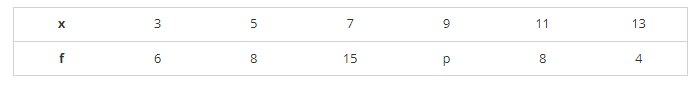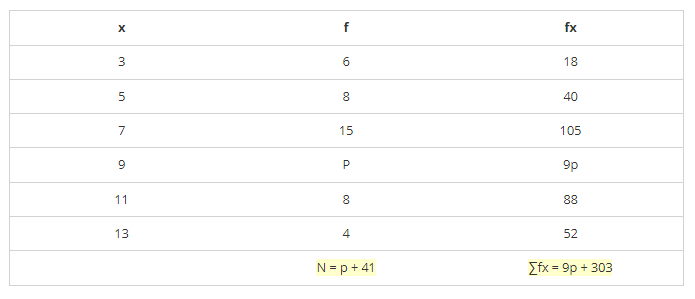# Find the missing frequency (p) for the following distribution whose mean is 7.68.

Question:

Find the missing frequency (p) for the following distribution whose mean is 7.68.Solution:It is given that,

Mean = 7.68

$\Rightarrow \sum f_{x} / N=7.68$

⇒ 9p + 303p + 41 = 7.68

⇒ 9p + 303 = 7.68p + 314.88

⇒ 9p − 7.68p = 314.88 − 303

⇒ 1.32p = 11.88

⇒ p = 11.881.32 = 9

⇒ p = 9

∴ p = 9.